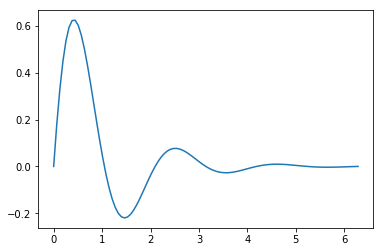Codex Vanitate archive

## So far this morning

Categories: Journal, Projects
Tags:
Published on: August 11, 2018

So far this morning I’ve

• Gotten jupyter notebooks running natively on my workstation rather than using the linux subsystem as a workaround
• Learned how to integrate functions in python using scipy
• Got exporting of jupyter notebooks to html working properly
• Built a scale model of the terrain around my home town in Unity
• Gained three levels on my warlock

Now I’m going to put my pants on.

## Integrating Functions

Quick example on integrating functions with jupyter, matplotlib, numpy, and scipy

### Import the required libraries

For this example we’ll be using $$f(x) = e^{-x}sin(3x)$$ as our function.

### Set the range for your plot and integration

To plot $$f(x)$$ we get an array of linearly spaced values from numpy, pass that array and our function to the plot function, and show the plot.

Evalating the integral $$\int_{0}^{2\pi}e^{-x}sin(3x)$$ simply requires passing our function and the range of the intgral to scipy’s quad function which will return a tuple of the integral and the estimated error.Integral: 0.29943976718048754
Estimated error 5.05015300411582e-13

page 1 of 1
Welcome , today is Saturday, July 11, 2020